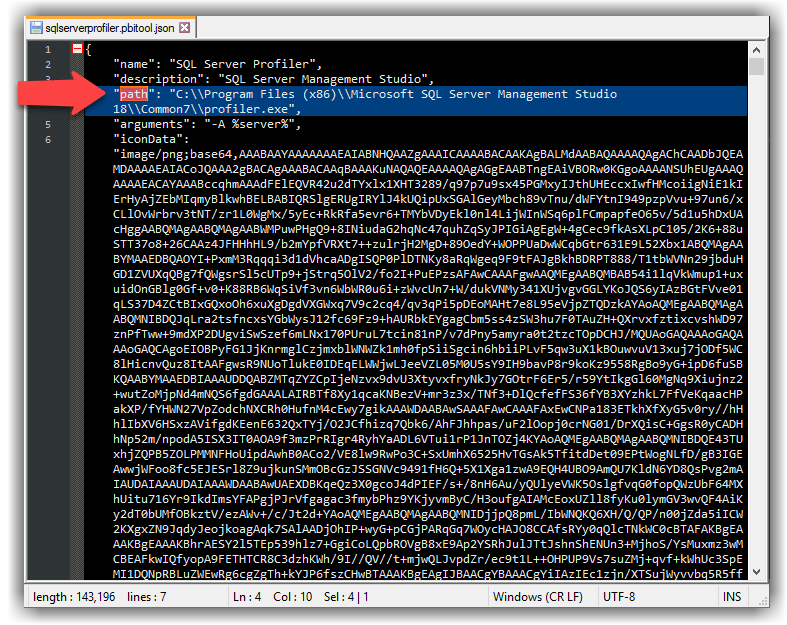There are some cases that we want to add a leading zero to a digit, such as showing 01 instead of 1, 02 instead of 2 and so on. We have two options to do this in Power BI, doing it in Power Query or doing it with DAX.

The first method is doing it in Power Query using the `Text.PadStart()` function.

Here is how the syntax of the function:

``Text.PadStart(text as nullable text, count as number, optional character as nullable text)``

And here is how the function works:

``Text.PadStart(input string, the length of the string, an optional character to be added to the beginning of the string util we reach to the string length)``

For example, `Text.PadStart("12345", 10 , "a")` returns `aaaaa12345` and `Text.PadStart("1", 2 , "0") `returns `01`.

Let’s create a list of integer values between 1 to 20 with the following expression:

``{1..20}``

Now we convert the list to a table by clicking the To Table button from the Transform tab:

Now we add a new column by clicking the Custom Column from the Add Column tab from the ribbon bar:

Now we use the following expression in the Custom Column window to pad the numbers with a leading zero:

## Quick Tips: Registering SQL Server Profiler as an External Tool in Power BI Desktop

It has been a long time that I use SQL Server Profiler to diagnose my data models in the Power BI Desktop. I wrote a blog post in June 2016 about connecting to the underlying Power BI Desktop model from different tools, including SQL Server Management Studio (SSMS), Excel and SQL Server Profiler. In this quick post, I share a pbitool.json file that you can use to register the SQL Server Profiler as an external tool. Read more about how to register an external tool here. This is quite handy as this way to use SQL Server Profiler to diagnose Power BI Desktop without needing to find the diagnostic port. As an external tool, the SQL Server Profiler automatically connects to the data model via the diagnostic port. You can download the sqlserverprofiler.pbitool.json file from here. After you download the file you can open it in a text editor to see or modify the JSON code. If you are using SSMS 18, then you do not even need to modify the file. If you use a different version, the only thing you have to change is the “path”.The contents of the sqlserverprofiler.pbitool.json file Continue reading “Quick Tips: Registering SQL Server Profiler as an External Tool in Power BI Desktop”

## Quick Tips: OData Feed Analyser Custom Function in Power Query

It’s been a while that I am working with OData data source in Power BI. One challenge that I almost always do not have a good understanding of the underlying data model. It can be really hard and time consuming if there is no one in the business that understands the underlying data model. I know, we can use `\$metadata` to get the metadata schema from the OData feed, but let’s not go there. I am not an OData expert but here is the thing for someone like me, I work with various data sources which I am not necessarily an expert in, but I need to understand what the entities are, how they are connected etc… then what if I do not have access any SMEs (Subject Matter Expert) who can help me with that?

So getting involved with more OData options, let’s get into it.

The custom function below accepts an OData URL then it discovers all tables, their column count, their row count (more on this later), number and list of related tables, number and list of columns of `type text`, `type number` and `Decimal.Type`.

``````// fnODataFeedAnalyser
(ODataFeed as text) =>
let
Source = OData.Feed(ODataFeed),
SourceToTable = Table.RenameColumns(
{{"Column1", "Name"}, {"Column2", "Data"}}
),
FilterTables = Table.SelectRows(
SourceToTable,
each Type.Is(Value.Type([Data]), Table.Type) = true
),
"Table Column Count",
each Table.ColumnCount([Data]),
Int64.Type
),
"Table Row Count",
each Table.RowCount([Data]),
Int64.Type
),
"Number of Related Tables",
each List.Count(Table.ColumnsOfType([Data], {Table.Type}))
),
"List of Related Tables",
each
if [Number of Related Tables] = 0 then
null
else
Table.ColumnsOfType([Data], {Table.Type}),
List.Type
),
ListOfRelatedTables,
"Number of Text Columns",
each List.Count(Table.SelectRows([Schema], each Text.Contains([Kind], "text"))[Name]),
Int64.Type
),
"List of Text Columns",
each
if [Number of Text Columns] = 0 then
null
else
Table.SelectRows([Schema], each Text.Contains([Kind], "text"))[Name]
),
"Number of Numeric Columns",
each List.Count(Table.SelectRows([Schema], each Text.Contains([Kind], "number"))[Name]),
Int64.Type
),
"List of Numeric Columns",
each
if [Number of Numeric Columns] = 0 then
null
else
Table.SelectRows([Schema], each Text.Contains([Kind], "number"))[Name]
),
"Number of Decimal Columns",
each List.Count(
Table.SelectRows([Schema], each Text.Contains([TypeName], "Decimal.Type"))[Name]
),
Int64.Type
),
"List of Decimal Columns",
each
if [Number of Decimal Columns] = 0 then
null
else
Table.SelectRows([Schema], each Text.Contains([TypeName], "Decimal.Type"))[Name]
),
#"Removed Other Columns" = Table.SelectColumns(
{
"Name",
"Table Column Count",
"Table Row Count",
"Number of Related Tables",
"List of Related Tables",
"Number of Text Columns",
"List of Text Columns",
"Number of Numeric Columns",
"List of Numeric Columns",
"Number of Decimal Columns",
"List of Decimal Columns"
}
)
in
#"Removed Other Columns"``````
Continue reading “Quick Tips: OData Feed Analyser Custom Function in Power Query”

## Quick Tips, Power BI Desktop, Query Parameters, Part 4, Passing Power Query Parameter Values to SQL Server Stored Procedures

I have written 3 blogposts about query parameters in the past.

This is the fourth one in the form of Quick Tips. Here is the scenario. One of my customers had a requirement to get data from a Stored Procedure from SQL Server. She required to pass the values from a Query Parameter back to SQL Server and get the results in Power BI.

The solution is somewhat easy. I created a simple stored procedure in AdventureWorksDW2019 as below:

``````CREATE PROCEDURE SP_Sales_by_Date
@date int
AS
BEGIN
SET NOCOUNT ON;
SELECT *
FROM [dbo].[FactInternetSales]
WHERE OrderDateKey >= @date
END
GO``````

In Power BI Desktop, get data from SQL Server, then:

• Enter Server name
• Enter Database name
• Select Data Connectivity Mode
``exec SP_Sales_by_Date @date = 20140101``